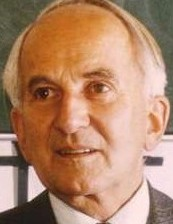# The Collatz ConjectureMathematical inquiry can lead down jagged paths hewed deeply in the landscape of abstract thought. The English cleric Charles Caleb Colton once said, “The study of mathematics, like the Nile, begins in minuteness but ends in magnificence.” This magnificence can be observed directly in the process of making a new discovery. Poised with creativity and a thorough knowledge, mathematicians often discover generalizations that transcend time as much as they do common understanding.

Sometimes, mathematicians can produce inquiries that are more recreational in nature. One such conundrum was raised by the German mathematician Lothar Collatz in 1937. Suppose I create a rule that if I have any even natural number, then I divide it by two, and if I have any odd natural number, then I multiply it by three and add one. More formally,$f(n) = n/2$ if$n$ is an even natural number, and$f(n)= 3n + 1$ if$n$ is an odd natural number. Collatz asserted that if one starts with any natural number$n$, and repeats the rule again and again, with each new appearing natural number, then the process will lead to the number one every time. For example, let’s start with the natural number 10. If I divide by two, I get 5. Taking 5 and multiplying by three and adding one, I get 16. Furthermore, if I notice that$16 = 2^4$, then I can divide by two four consecutive times to come to the natural number one as the Collatz Conjecture (as it is originally named) proposes.

There was a power of two in the last step of the example.  It is easily seen that the conjecture is true for any power of two, since all powers of two are even and dividing them by their power number of times by two leads to one. This could spark another question that is equivalent: Starting with any natural number $n$ does repeating the rule always lead to a power of two? The simplicity of such a question can be very deceiving in the world of mathematics.

The prolific mathematician, Paul Erdős, in speaking of the Collatz Conjecture, once said, “Mathematics is not ready for such problems.” He actually offered \$500 dollars for its solution (which is written about here). I agree with Erdős. Mathematics is not necessarily equipped at this time to handle such inquiries. However, it could be interesting to consider the existence of a counterexample. Can you show one? What would one look like? Would it lead to any contradicitons? These are the type questions that spark a journey down the paths of mathematical inquiry and lead to unexpected solutions.Avery Carr is a senior analyst and past senior editor for the American Mathematical Society Grad Blog. He and his wife, Alison, live in Olive Branch, MS.
This entry was posted in AMS, General, Math, Math in Pop Culture, Mathematics in Society, Uncategorized. Bookmark the permalink.

### 3 Responses to The Collatz Conjecture

1.Daniel Zardo says:

Dear Avery.
I thought of an approach using probability, which I describe here:
http://www.therealebook.com/Collatz/Collatz.html
Would appreciate if you could take a look and share your thoughts about it.
2.Reza says:
3.Kamal barghout says: